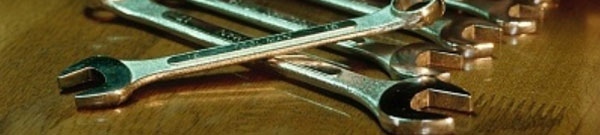# Characteristics of DC (Direct Current) series motor(1)Relationship between starter speed, torque and current

A circuit of a motor basically consists of only coils. The resistance value inside the circuit is very small because only the resistance value of the coil exists in the circuit. According to Ohm’s law, the current value increases extremely when the battery voltage (12 V) is constant and the resistance value is small. As a result, large amount of current flows to the starter, and the maximum torque is produced just after the starter begins to turn.

Since the motor and the generator have similar construction, reverse direction voltage (counter-electromotive force) is generated when the motor revolves, interfering with the current to flow smoothly.

Since the counter-electromotive force become larger as the starter speed increases, current flowing through the motor becomes smaller, and the torque and the current value decrease.

The gear ratio of the ring gear to the starter pinion gear is approx. 1:10 to 1:15.

Output is low just after the starter begins to turn because the torque is large and the starter speed is low, but the output increases to the maximum output point in accordance with the change in torque and starter speed, and then it decreases. Output follows the curved line as shown in the figure in accordance with the change in torque and starter speed.

(2)Relationship between current and voltage

When the starter starts, the battery terminal voltage decreases due to a large amount of current flow. When a large amount of current flows, the internal resistance of the battery cannot be neglected. According to Ohm’s law, voltage drop is greater when the current value is larger. Voltage drop is reduced as the flowing amount of current decreases, and the battery voltage returns to the normal value.